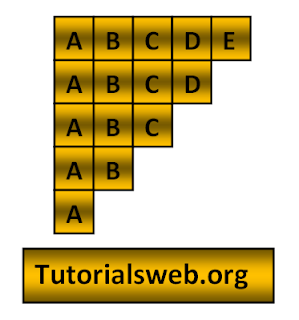## Write "Java"  Program to print below Alphabet Pattern14 (or) Character Pattern14:To understand this example, you should have knowledge of the following topics:

·   Java programming operators.

Java Character pattern 14 program using For loop:
`import java.util.Scanner;`
`class alphabetPattern14`
`{`
`public static void main(String[] ags)`
`{`
`    int i, j,k;`
`   System.out.println("Enter the number of lines");`
`   Scanner s = new Scanner(System.in);`
`   k = s.nextInt();`
`    for(i = 1;i <= k;i++)`
`    {  `
`        for(j = 1;j <= k-i+1;j++)`
`           {`
`            System.out.print((char)(j+64));`
`            }`
`        System.out.println();`
`    }`
`  }`
Output:
```
Enter the number of lines
5
ABCDE
ABCD
ABC
AB
A
```
Explanation:
• First three integers "i", "j", "k"  are declared of type int.
• Then in the First for loop “i” value is initialized with a value of "1".
• Now “i” value is checked with the condition "i <= k"(where "k" value is given through Keyboard). If the condition is True loops enter into second for loop.
• Now the loop enters into second for loop and checks the condition "j <= k-i+1". Where "j = 1". and the condition is True.
• Now prints the " Alphabet". Print up to second for loop condition becomes False.
• When the value of "i"  becomes less than "k" value the program terminates.

## Search This Blog

### C Data Types and Types of data types in C

Data types specify the type of data that a variable can store. All C compilers support Five fundamental data types, they are an integer(int...